• ## C++数据结构——栈

万次阅读 多人点赞 2018-06-25 21:54:49
C++数据结构——栈 最近计划再复习一遍数据结构，看到一篇博客：https://www.cnblogs.com/QG-whz/p/5170418.html#_label0。 1、栈(Stack)是一种线性存储结构，它具有如下特点： ...
                                                      C++数据结构——栈

最近计划再复习一遍数据结构，看到一篇博客：https://www.cnblogs.com/QG-whz/p/5170418.html#_label0。
1、栈(Stack)是一种线性存储结构，它具有如下特点：
（1）栈中的数据元素遵守“先进后出"(First In Last Out)的原则，简称FILO结构。（后进先出的叫法，也是可以的）
（2）限定只能在栈顶进行插入和删除操作。
2、栈的相关概念：
（1）栈顶与栈底：允许元素插入与删除的一端称为栈顶，另一端称为栈底。
（2）压栈：栈的插入操作，叫做进栈，也称压栈、入栈。
（3）弹栈：栈的删除操作，也叫做出栈。
3、栈的常用操作为：
（1）弹栈，通常命名为pop
（2）压栈，通常命名为push
（3）求栈的大小
（4）判断栈是否为空
（5）获取栈顶元素的值
4、栈的常见分类：
（1）基于数组的栈——以数组为底层数据结构时，通常以数组头为栈底，数组头到数组尾为栈顶的生长方向
（2）基于单链表的栈——以链表为底层的数据结构时，以链表头为栈顶，便于节点的插入与删除，压栈产生的新节点将一直出现在链表的头部
5、实例分析
使用标准库的栈时, 应包含相关头文件，在栈中应包含头文件： #include< stack > 。定义：stack< int > s;
s.empty();         //如果栈为空则返回true, 否则返回false;
s.size();          //返回栈中元素的个数
s.top();           //返回栈顶元素, 但不删除该元素
s.pop();           //弹出栈顶元素, 但不返回其值
s.push();          //将元素压入栈顶
（1）基于数组的栈
#include <stack>
#include <iostream>
using namespace std;

int main()
{
stack<int> mystack;
int sum = 0;
for (int i = 0; i <= 10; i++){
mystack.push(i);
}
cout << "size is " << mystack.size() << endl;
while (!mystack.empty()){
cout << " " << mystack.top();
mystack.pop();
}
cout << endl;
system("pause");
return 0;
}
//size is 11
// 10 9 8 7 6 5 4 3 2 1 0
（2）基于单链表的栈
#include <iostream>
using namespace std;
template<class T>class Stack
{
private:
struct Node
{
T data;
Node *next;
};
Node *p;
int length;

public:
Stack()
{
length = 0;
}
void push(T n)//入栈
{
Node *q = new Node;
q->data = n;
{
p = q;
}
else
{
q->next = p;
p = q;
}
length++;
}

T pop()//出栈并且将出栈的元素返回
{
if (length <= 0)
{
abort();
}
Node *q;
T data;
q = p;
data = p->data;
p = p->next;
delete(q);
length--;
return data;
}
int size()//返回元素个数
{
return length;
}
T top()//返回栈顶元素
{
return p->data;
}
bool isEmpty()//判断栈是不是空的
{
if (length == 0)
{
return true;
}
else
{
return false;
}
}
void clear()//清空栈中的所有元素
{
while (length > 0)
{
pop();
}
}
};
int main()
{
Stack<char> s;
s.push('a');
s.push('b');
s.push('c');
while (!s.isEmpty())
{
cout << s.pop() << endl;
}
system("pause");
return 0;
}

练习1、实现一个特殊的栈，在实现栈的基本功能的基础上，再实现返回栈中最小元素的操作。
解法参考博客：https://blog.csdn.net/cherrydreamsover/article/details/79475925，具体过程如下：
（1）使用两个栈，一个栈用来保存当前的元素，记做：stackData，一个栈用来保存压入操作每一步的最小元素，记做：stackMin。
（2）入栈：当stackData栈中压入一个数据时，判断satckMin中是否为空。若为空，将该元素压入stackMin栈中。若不空，判断两者之间的大小，当前者小于或等于后者时，将前者中的数据压入后者中；当前者大于后者时，
不进行任何操作。
（3）出栈：保证stackMin中栈顶的元素是stackData中最小的。
#include<iostream>
#include <stack>
#include <cassert>
using  namespace std;

//方法一：  一个辅助栈，如果这个栈为空，直接将元素入这个栈，如果辅助栈中有元素，将压入的元素和辅助栈顶元素比较，
//压入两者中较小的那个元素使得辅助栈总是维持栈顶元素为最小值。
//class Stack
//{
//public:
//  void Push(int data)
//  {
//      stackData.push(data);
//      if (stackMin.empty())
//      {
//          stackMin.push(data);
//      }
//      else
//      {
//          int tmp = stackMin.top();
//          int min = data > tmp ? tmp : data;
//          stackMin.push(min);
//      }
//  }
//
//  void Pop()
//  {
//      assert(!stackData.empty() && !stackMin.empty());
//      stackData.pop();
//      stackMin.pop();
//  }
//
//  int GetMin()
//  {
//      assert(!stackMin.empty());
//      return stackMin.top();
//  }
//
//private:
//  stack<int> stackData;
//  stack<int> stackMin;
//};

//方法二： 一个辅助栈，如果这个栈为空，直接将元素入这个栈，如果辅助栈中有元素，将压入的元素和辅助栈顶元素比较，
//如果压入的元素小于等于辅助栈顶元素，者将这个元素入辅助栈，否则无操作，出栈的时候判断要出栈的元素是否等于辅助
//栈顶元素，如果是，也将辅助栈顶元素出栈。否则无操作。
class Stack
{
public:
void Push(int data)
{
stackData.push(data);
if (stackMin.empty())
{
stackMin.push(data);
}
else
{
if (data <= stackMin.top())
{
stackMin.push(data);
}
}
}

void Pop()
{
assert(!stackData.empty() && !stackMin.empty());
if (stackData.top() == stackMin.top())
{
stackMin.pop();
}
stackData.pop();
}

int GetMin()
{
assert(!stackMin.empty());
return stackMin.top();
}

private:
stack<int> stackData;
stack<int> stackMin;

};

int main()
{
Stack s;
//s.Push(5);
s.Push(36);
s.Push(15);
s.Push(95);
s.Push(50);
s.Push(53);
cout << s.GetMin() << endl;
system("pause");
return 0;
}//15
（3）栈的应用举例
1）进制转换
2）括号匹配的检验
3）行编辑程序
4）迷宫求解、汉诺塔等经典问题
5）表达式求值
6）栈与递归的实现

练习2、剑指offer面试题30——包含min函数的栈
题目描述

定义栈的数据结构，请在该类型中实现一个能够得到栈最小元素的min函数。

class Solution {
public:
stack<int> stackData;//保存数据用的栈stackData
stack<int> stackMin;//保存最小的数的栈stackMin，其中它的栈顶始终为最小的数
void push(int value) {
stackData.push(value);
if(stackMin.empty())
stackMin.push(value);//如果stackMin为空，则value是最小的值，入栈
else{
if(stackMin.top()>=value)
stackMin.push(value);//否则当value小于等于stackMin的栈顶元素时，入栈（等于的时候也入栈是因为我考虑有相同的数）
}
}
void pop() {
if(stackData.top()==stackMin.top())//如果出栈的数刚好是最小的数，那么stackMin也应该出栈
stackMin.pop();
stackData.pop();
}
int top() {
return stackData.top();//栈顶元素应返回stackData的栈顶元素
}
int min() {
return stackMin.top();//stackMin的栈顶元素即是最小的数
}
};
运行结果：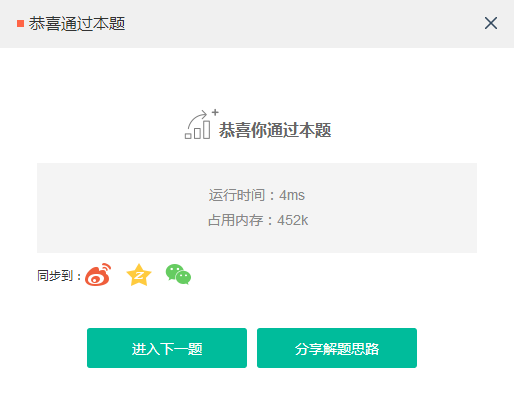练习3、剑指offer面试题31——栈的压入、弹出序列
参考博客：https://blog.csdn.net/budf01/article/details/76232497，解题思路为：创建一个栈进行压入、弹出操作。具体操作如下：  （1）当栈为空或者栈顶元素和popV当前元素不相等时，将pushV当前元素压入；  （2）当栈顶元素与popV当前元素相等时，将栈顶元素弹出，并移至popV下一个元素；  （3）如果需要压入的元素个数大于pushV的元素个数，说明popV不可能是pushV的弹出序列。
代码为：
class Solution {
public:
bool IsPopOrder(vector<int> pushV,vector<int> popV) {
//特殊输入测试
if(pushV.empty() || popV.empty() || pushV.size()!=popV.size())
return false;
stack<int> mystack;//定义一个辅助栈
int index=0;
for(int i=0;i<popV.size();i++){
//当辅助栈为空或者栈顶元素和popV当前元素不相等时，将pushV当前元素压入
while(mystack.empty()||mystack.top()!=popV[i]){
if(index>=pushV.size())
//如果需要压入的元素个数大于pushV的元素个数，说明popV不可能是pushV的弹出序列
return false;
mystack.push(pushV[index++]);
}
//当栈顶元素与popV当前元素相等时，将栈顶元素弹出，并移至popV下一个元素
if(mystack.top()==popV[i])
mystack.pop();
}
return true;
}
};
运行结果：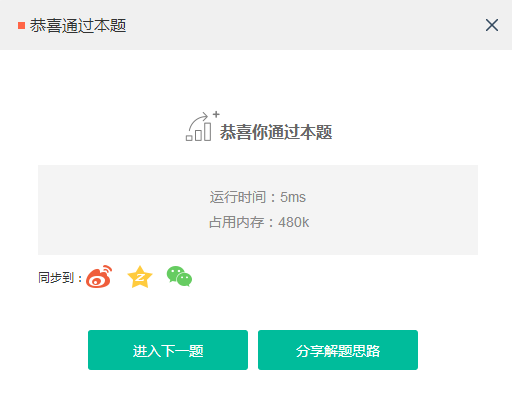当然可以利用其他思想解决，如引入哈希或直接利用向量的方式求解。
class Solution {
public:
bool IsPopOrder(vector<int> pushV,vector<int> popV) {
if(pushV.empty() && popV.empty() && pushV.size() != popV.size()){
return false;
}
map<int,int> Hash; //用map做一个映射，入栈顺序的值不一定是递增
for(int i=0;i<pushV.size();i++){
Hash[pushV[i]]=i+1;
}
int now=Hash[popV]; //当前最靠后入栈的键值，例如题目给的4 3 5 1 2，now先等于4，再等于5
for(int i=0;i<popV.size();i++){
//如果入栈序列中没有这个值
if(Hash[popV[i]]==0){
return false;
}
if(Hash[popV[i]]>=now){
now=Hash[popV[i]];
}
else if(Hash[popV[i]]<=Hash[popV[i-1]]){
continue ;
}
else{
return false;
}
}
return true;
}
};
练习4、简单的括号匹配判断
例如，爱奇艺的一道实习在线编程题：当输入为（）（（））（），返回true；当输入为）（）（）（）（）），返回false，时间15min。（不能使用栈）
1、假设可以使用栈（15min可以完成）
C++代码：
#include <iostream>
#include <stack>
#include <vector>
using namespace std;

bool isRight(vector<char> &vec){
stack<char> stack1;
bool index = false;
if (vec.size() <= 1 || vec!='(' || vec.size()%2!=0){
return index;
}
for (int i = 0; i < vec.size(); i++){
if (vec[i] == '(')
stack1.push(vec[i]);
else if (vec[i] == ')')
stack1.pop();
}
if (stack1.empty())
index = true;
return index;
}

int main(){
//输入不定长的括号
vector<char> vec;
char tmpCh;
char t;
cout << "输入一串括号为：";
do{
cin >> tmpCh;
vec.push_back(tmpCh);
} while ((t = cin.get()) != '\n');

//调用isRight函数
bool myRes = isRight(vec);
cout << myRes << endl;
system("pause");
return 0;
}
运行结果：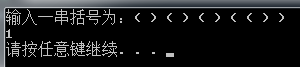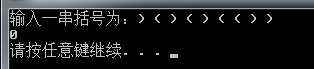python代码：
def isRight(str1):
index = False
tmp = []
if(len(str1)>=2 and len(str1)%2==0 and str1=='('):
for id in range(len(str1)):
if str1[id] == '(':
tmp.append(str1[id])
else:
tmp.pop()
if len(tmp)==0:
index = True
return index

if __name__ == "__main__":
try:
while True:
str1 = [i for i in input().split()]
print(isRight(str1))  # 返回判断结果
except:
pass
运行结果：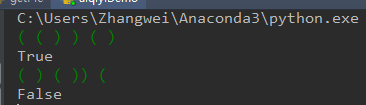以下是我的想法，具体的过程如下：
（1）由于不能使用栈，将左括号定义为数值1，右括号定义为数值-1，存放到向量id（C++）或列表tmp （Python）中；
（2）初始化变量sum，用于判断总的求和结果是否等于0，若不等于0，则肯定不正确，若等于0，不一定正确；
（3）循环遍历输入的括号向量vec，判断当前括号属性的同时，进行累加求和，如果求和值小于等于-1，break（跳出循环）；
（4）最后再检查sum是否等于0，此时若等于0，则为正确。
C++代码：
#include <iostream>
#include <vector>
using namespace std;

bool isRight(vector<char> &vec){
vector<int> id(vec.size()); //用于存放左右括号的属性：左括号用1表示，右括号用-1表示
int sum = 0;
bool index = false;
if (vec.size() <= 1 || vec!='(' || vec.size()%2!=0){
return index;
}
for (int i = 0; i < vec.size(); i++){
if (vec[i] == '('){
id.push_back(1);
sum = id[i] + sum;
}

else if (vec[i] == ')'){
id.push_back(-1);
sum = id[i] + sum;
if (sum <= -1)
break;
}
}

if (sum == 0)
index = true;
return index;
}

int main(){
//输入不定长的括号
vector<char> vec;
char tmpCh;
char t;
cout << "输入一串括号为：";
do{
cin >> tmpCh;
vec.push_back(tmpCh);
} while ((t = cin.get()) != '\n');

//调用isRight函数
bool myRes = isRight(vec);
cout << myRes << endl;
system("pause");
return 0;
}
运行结果同上
python代码：
def isRight(str1):
index = False
sum = 0
tmp = []
if(len(str1)>=2 and len(str1)%2==0 and str1=='('):
for id in range(len(str1)):
if str1[id] == '(':
tmp.append(1)
sum += tmp[id]
else:
tmp.append(-1)
sum += tmp[id]
if sum<=-1:
break
if sum == 0:
index = True
return index

if __name__ == "__main__":
try:
while True:
str1 = [i for i in input().split()]
print(isRight(str1))  # 返回判断结果
except:
pass
运行结果同上。
展开全文• ## C++数据结构——队列

万次阅读 多人点赞 2018-06-26 22:20:30
C++数据结构——队列参考博客：http://www.cnblogs.com/QG-whz/p/5171123.htmlhttp://www.169it.com/article/2718050585107790752.html1、队列（Queue）与栈一样，是一种线性存储结构，它具有如下特点：（1）队列中...
                                                  C++数据结构——队列

参考博客：
数据结构图文解析之：队列详解与C++模板实现 C++ stl队列Queue用法介绍:删除，插入等操作代码举例

1、队列（Queue）与栈一样，是一种线性存储结构，它具有如下特点：
（1）队列中的数据元素遵循“先进先出”（First In First Out）的原则，简称FIFO结构；
（2）在队尾添加元素，在队头删除元素。
2、队列的相关概念：
（1）队头与队尾： 允许元素插入的一端称为队尾，允许元素删除的一端称为队头；
（2）入队：队列的插入操作；
（3）出队：队列的删除操作。
3、队列的操作：
（1）入队： 通常命名为push()
（2）出队： 通常命名为pop()
（3）求队列中元素个数
（4）判断队列是否为空
（5）获取队首元素
4、队列的分类：
（1）基于数组的循环队列（循环队列）
（2）基于链表的队列（链队列）
5、实例分析
C++队列queue模板类的定义在<queue>头文件中,queue 模板类需要两个模板参数，一个是元素类型，一个容器类型，元素类型是必要的，容器类型是可选的，默认为deque 类型。C++队列Queue是一种容器适配器，它给予程序员一种先进先出(FIFO)的数据结构。
那么我们如何判断队列是空队列还是已满呢？
a、栈空： 队首标志=队尾标志时，表示栈空。
b、栈满 : 队尾+1 = 队首时，表示栈满。
使用标准库的队列时, 应包含相关头文件，在栈中应包含头文件： #include< queue> 。定义：queue< int > q;
q.empty()               如果队列为空返回true，否则返回false
q.size()                返回队列中元素的个数
q.pop()                 删除队列首元素但不返回其值
q.front()               返回队首元素的值，但不删除该元素
q.push()                在队尾压入新元素
q.back()                返回队列尾元素的值，但不删除该元素

（1）基于数组的循环队列（循环队列）
以数组作为底层数据结构时，一般讲队列实现为循环队列。这是因为队列在顺序存储上的不足：每次从数组头部删除元素（出队）后，需要将头部以后的所有元素往前移动一个位置，这是一个时间复杂度为O（n）的操作。具体的示例图参考：http://www.cnblogs.com/QG-whz/p/5171123.html。
循环队列，可以把数组看出一个首尾相连的圆环，删除元素时将队首标志往后移动，添加元素时若数组尾部已经没有空间，则考虑数组头部的空间是否空闲，如果是，则在数组头部进行插入。参考博客：【c++版数据结构】之循环队列的实现，判断循环队列是“空”还是“ 满”，有两种处理方法：
A. 设置状态标志位以区别空还是满B. 少用一个元素，约定“队头front在队尾rear的下一个位置（指的是环的下一个位置）”作为“满”的标志
C语言中，不能用动态分配的一维数组来实现循环队列，如果用户的应用程序中设有循环队列，则必须为它设定一个最大队列长度；如果用户无法预估所用队列的最大长度，则宜采用链队列。
定义front为队列头元素的位置，rear为队列尾元素的位置，MAXSIZE为循环队列的最大长度。注意以下几点，循环队列迎刃而解：
A.  求元素的个数：(rear - front + MAXSIZE) % MAXSIZEB.  front/rear指向逻辑的下一个空间  front =（front+1)%MAXSIZE，rear = (rear+1)%MAXSIZEC.  判空：front == rearD.  判满：(rear+1+MAXSZIE) == front

例子1、简单的队列操作
#include <queue>
#include <iostream>
using namespace std;

int main(){
queue<int> q;
for (int i = 0; i < 10; i++){
q.push(i);
}
if (!q.empty()){
cout << "队列q非空！" << endl;
cout << "q中有" << q.size() << "个元素" << endl;
}
cout << "队头元素为：" << q.front() << endl;
cout << "队尾元素为：" << q.back() << endl;
for (int j = 0; j < 10; j++){
int tmp = q.front();
cout << tmp << " ";
q.pop();
}
cout << endl;
if (!q.empty()){
cout << "队列非空！" << endl;
}
system("pause");
return 0;
}

运行结果：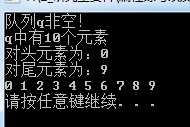例子2、循环队列的C++实现

#include <iostream>
#include <queue>
#include <string>
using namespace std;

template <typename T>
class LoopQueue
{
public:
LoopQueue(int c = 10);
~LoopQueue();
bool isEmpty();        //队列的判空
int size();            //队列的大小
bool push(T t);        //入队列
bool pop();            //出队列
T front();            //队首元素

private:
int capacity;
int begin;
int end;
T*  queue;
};

template<typename T>
LoopQueue<T>::LoopQueue(int c = 10)
:capacity(c), begin(0), end(0), queue(nullptr)
{
queue = new T[capacity];
};

template<typename T>
LoopQueue<T>::~LoopQueue()
{
delete[]queue;
}

template <typename T>
bool LoopQueue<T>::isEmpty()                   //判断循环队列是否为空
{
if (begin == end)
return true;
return false;
};

template<typename T>
int LoopQueue<T>::size()
{
return (end - begin + capacity) % capacity; //计算循环队列的长度
};

template<typename T>
bool LoopQueue<T>::push(T t)
{
if (end + 1 % capacity == begin)            //判断队列是否已满
{
return false;
}
queue[end] = t;
end = (end + 1) % capacity;
return true;
};

template <typename T>
bool LoopQueue<T>::pop()                        //判断队列是否为空
{
if (end == begin)
{
return false;
}
begin = (begin + 1) % capacity;
return true;
};

template <typename T>
T LoopQueue<T>::front()
{
if (end == begin)
{
return false;
}
return queue[begin];
};

int main()
{
LoopQueue<string> queue(6);
queue.push("one");
queue.push("two");
queue.push("three");
queue.push("four");
queue.push("five");
cout << "队列长度" << queue.size() << endl;
while (!queue.isEmpty())
{
cout << queue.front() << endl;
queue.pop();
}
getchar();
//system("pause");
return 0;
}
运行结果：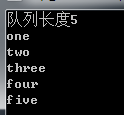（2）基于链表的队列（链队列）
链队列是基于链表实现的队列，它不存在数组的O(n)的元素移动问题或空间浪费问题。我们所要确定的就是链表哪头做队首，哪头做队尾。显然我们应该以链表头部为队首，链表尾部为队尾。存储一个指向队尾的指针，方便从链表尾插入元素；使用带头节点的链表，方便从链表头删除元素。
代码参考：链式队列的C++实现
#include <iostream>
#include <cstdlib>
using namespace std;

struct QNode    //定义队列结点的数据结构
{
QNode *next; //指针域,指向下一个结点
double data;    //数据域，存储队列信息
};

{
QNode *front;      //队首指针,指向QNode类型的指针
QNode *rear;       //队尾指针
};

{
QNode *q;
q = new QNode;    //申请一个结点的空间
q->next = NULL;   //当作头结点
//队首与队尾指针都指向这个结点，指针域为NULL
Q.front = q;
Q.rear = q;
}

{
if (Q.rear == Q.front)
return 0;
else
return 1;
}

void EnQueue(LinkQueue &Q, double e)     //从队列尾部插入元素
{
QNode *p;    //新创建一个结点
p = new QNode;
p->next = NULL;
p->data = e;  //输入数据信息
//将新结点插入队列尾部
Q.rear->next = p;
Q.rear = p;       //设置新的尾结点
}

void DeQueue(LinkQueue &Q, double &e)   //从队列首部删除一个结点
{
QNode *p;
p = Q.front->next;
e = p->data;    //保存要出队列的数据
Q.front->next = p->next;       //将下一个结点当作头结点后面链接的第一个结点
if (Q.rear == p)    //如果要删除的元素即为尾结点，则将头指针赋予尾指针，一同指向头结点，表示队列为空
Q.rear = Q.front;
delete p;
}

{
while (Q.front)
{
Q.rear = Q.front;    //从头节点开始，一个一个删除队列结点，释放空间
delete Q.front;
Q.front = Q.rear;
}
}
int main()
{
InitQueue(*Q);
cout << "开始往队列里输入数据，以-1作为结束符" << endl;
cout << "请输入一个数：" << endl;
double a, x;
cin >> a;
while (a != -1)
{
EnQueue(*Q, a);
cout << "请输入一个数：" << endl;
cin >> a;
}
//输出队列元素,队首->队尾
QNode *p;
p = Q->front->next;
if (p == NULL)     //如果为空表，直接退出
{
cout << "队列为空！" << endl;
return 0;
}
cout << "队列数据依次为：" << endl;
while (p != NULL)
{
cout << p->data << " ";
p = p->next;
}
cout << endl;
//删除队列元素
while (!IsEmpty(*Q))
{
DeQueue(*Q, x);
cout << x << " ";
}
//释放内存空间
delete Q->front;
delete Q;
system("pause");
return 0;
}
运行结果：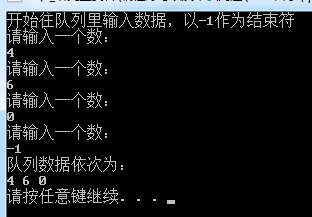还可以参考代码：C++ 链队列和循环队列基本操作。

总结：
1、循环队列中判断队空的方法是判断front==rear，队满的方法是判断front=（rear+1）%MAXSIZE。（为什么不用一个length表示队长，当length==maxSize时表示队满，原因就是，在频繁的队列操作中，多出一个变量会大量的增加执行时间，所以不如浪费一个数组空间来得划算。）
2、用单链表表示的链式队列特别适合于数据元素变动较大的情形，而且不存在溢出的情况。
展开全文循环队列 链队列
• c++数据结构,c++,数据结构,c++数据结构,c++,数据结构c++ 数据结构
• 数据 数据结构 c++数据结构 c++ 数据结构 c++ 数据结构 数据 数据结构 c++数据结构 c++ 数据结构 数据 数据结构 c++数据结构 c++ 数据结构 数据 数据结构 c++数据结构
• 严蔚敏c++数据结构c++严蔚敏c++数据结构严蔚敏c++数据结构c++严蔚敏c++数据结构严蔚敏c++数据结构c++严蔚敏c++数据结构
• C++数据结构 C++数据结构 C++数据结构 C++数据结构 C++数据结构 C++数据结构 C++数据结构 C++数据结构 C++数据结构
• C_C++数据结构基础.docx C_C++数据结构基础.docx C_C++数据结构基础.docx C_C++数据结构基础.docx
• ## c++数据结构总结（干货）

千次阅读 多人点赞 2020-05-23 13:57:59
C++数据结构实用干货！！看到就是赚到！
作为一个程序员以及技术小白，掌握c++中的数据结构必不可少，本人长期混迹于CSDN，这里面有很多大佬，做的关于数据结构的总结特别深入详细。
在这里总结了自己平时阅读过程中收藏的关于数据结构觉得很优秀的博文（总阅读量100W+），和大家一起学习进步！工欲善其事，必先利其器！（收藏一下，少走弯路）

array 传送门哈希表 传送门链表 传送门vector 传送门map 传送门（优秀）unordered_map 传送门红黑树 传送门栈（超强详解） 传送门队列（干货） 传送门set 传送门map和unordered_map的区别 传送门
展开全文• c++数据结构 算法模板 c++数据结构 算法模板 c++数据结构 算法模板 c++数据结构 算法模板 c++数据结构 算法模板c++数据结构 算法模板c++数据结构 算法模板
• C++数据结构与算法（第四版）C++数据结构与算法（第四版）
• c++ 数据结构 算法 数据结构与算法 编程 c++ 数据结构 算法 数据结构与算法 编程 c++ 数据结构 算法 数据结构与算法 编程 c++ 数据结构 算法 数据结构与算法 编程 还用我说嘛！！！？？？
• 最优二叉树 c++ 数据结构 最优二叉树 c++ 数据结构 最优二叉树 c++ 数据结构
• C++数据结构--清华大学C++数据结构--清华大学C++数据结构--清华大学C++数据结构--清华大学C++数据结构--清华大学C++数据结构--清华大学C++数据结构--清华大学
• c++数据结构实验指导书c++数据结构实验指导书c++数据结构实验指导书c++数据结构实验指导书
• c++数据结构.rarc++数据c++数据结c++数据结构.rar构.rar结构.rarc++数据结构.rar
• <<C++数据结构>> C++数据结构原理与经典问题求解》是一部关于计算机科学与工程领域基础性核心课程——数据结构与算法的专著，本书《内容实用，体例新颖，结构清晰，既可以作为大、中专院校在校师生相关课程的参考...
• C++数据结构与算法实验报告C++数据结构与算法实验报告C++数据结构与算法实验报告 代码在报告里面代码
• C++数据结构——链表参考博客：（1）实践：https://www.cnblogs.com/renyuan/archive/2013/05/21/3091524.html（2）实践：https://blog.csdn.net/lg1259156776/article/details/47021505（3）理论：数据结构（二）...

C++数据结构——链表

参考博客：
（1）实践：https://www.cnblogs.com/renyuan/archive/2013/05/21/3091524.html
（2）实践：https://blog.csdn.net/lg1259156776/article/details/47021505
（3）理论：数据结构（二）链表、链队列https://blog.csdn.net/lg1259156776/article/details/47018813
（4）理论：https://blog.csdn.net/lg1259156776/article/details/46993591
（5）官网教程：http://www.cplusplus.com/reference/list/list/
1、线性表的简介
线性表是一种线性结构，它是由零个或多个数据元素构成的有限序列。线性表的特征是在一个序列中，除了头尾元素，每个元素都有且只有一个直接前驱，有且只有一个直接后继，而序列头元素没有直接前驱，序列尾元素没有直接后继。数据结构中常见的线性结构有数组、单链表、双链表、循环链表等。线性表中的元素为某种相同的抽象数据类型。可以是C语言的内置类型或结构体，也可以是C++自定义类型。
2、数组
数组在实际的物理内存上也是连续存储的，数组有上界和下界。C语言中定义一个数组：数组下标是从0开始的，a对应第一个元素。其中，a称为数组a的下界，a[n]称为数组a的上界。超过这个范围的下标使用数组，将造成数组越界错误。数组的特点是：数据连续，支持快速随机访问。数组分为固定数组与动态数组。其中固定数组的大小必须在编译时就能够确认，动态数组允许在运行时申请数组内存。复杂点的数组是多维数组，多维数组实际上也是通过一维数组来实现的。在C语言中，可以通过malloc来分配动态数组，C++使用new。另外，C++的标准模板库提供了动态数组类型vector以及内置有固定数组类型array。
3、链表的分类（有篇博客总结得很全面：https://blog.csdn.net/baweiyaoji/article/details/76071053）
（1）单向链表
（2）单向循环链表
（3）双向链表
（4）双向循环链表
4、单向链表
单向链表是链表的一种。链表由节点所构成，节点内含一个指向下一个节点的指针，节点依次链接成为链表。因此，链表这种数据结构通常在物理内存上是不连续的。链表的通常含有一个头节点，头节点不存放实际的值，它含有一个指针，指向存放元素的第一个节点。
5、单向循环链表
单向循环链表是另一种形式的链式存储结构，其特点是表中最后一个结点的指针域指向头结点，整个链表形成一个环。由此，从表中的任一结点出发均可找到表中的其他结点。
6、双向链表
以上讨论的链式存储结构的特点只有一个指示直接后继的指针域，由此，从某个结点出发只能顺着指针往后寻找其他结点。若要寻找结点的直接的前驱，则需从表头指针出发。换句话说，在单链表中，NextElem的执行时间为O（1），而PriorElem的执行时间为O（n）。为克服单链表这种单向性的缺点，可利用双向链表。
双向链表的每个结点除含有数据域外，还有两个指针域，分别指向直接前驱结点和直接后继结点。因此，从双向链表中的任一结点开始，均可方便地访问其前驱结点和后继结点。具体见博客：
https://blog.csdn.net/mianhuantang848989/article/details/72393561
7、双向循环链表

https://blog.csdn.net/mianhuantang848989/article/details/72393561

8、实例分析
若使用标准库，需添加#include <list>，下面是常见一些操作：
assign() 给list赋值
back() 返回最后一个元素
begin() 返回指向第一个元素的迭代器
clear() 删除所有元素
empty() 如果list是空的则返回true
end() 返回末尾的迭代器
erase() 删除一个元素
front() 返回第一个元素
get_allocator() 返回list的配置器
insert() 插入一个元素到list中
max_size() 返回list能容纳的最大元素数量
merge() 合并两个list
pop_back() 删除最后一个元素
pop_front() 删除第一个元素
push_back() 在list的末尾添加一个元素
push_front() 在list的头部添加一个元素
rbegin() 返回指向第一个元素的逆向迭代器
remove() 从list删除元素
remove_if() 按指定条件删除元素
rend() 指向list末尾的逆向迭代器
resize() 改变list的大小
reverse() 把list的元素倒转
size() 返回list中的元素个数
sort() 给list排序
splice() 合并两个list
swap() 交换两个list
unique() 删除list中重复的元素
（1）表的基本操作
#include <list>
#include <iostream>
using namespace std;

int main(){
//list<int> L1{4,2,6,5};
list<int> L1;
list <int>::iterator L1_iter;
L1.push_back(4);
L1.push_back(2);
L1.push_back(6);
L1.push_back(5);

L1.reverse();//反转
for (L1_iter = L1.begin(); L1_iter != L1.end();L1_iter++){
cout << " " << *L1_iter;
}
cout << endl;
system("pause");
return 0;
}
运行结果：
 5 6 2 4
（2）剑指offer面试题24——反转链表
/*
struct ListNode {
int val;
struct ListNode *next;
ListNode(int x) :
val(x), next(NULL) {
}
};*/
class Solution {
public:
ListNode* pPrev = nullptr;

while(pNode!=nullptr){//输入的链表不为空
ListNode* pNext = pNode->next;
if(pNext == nullptr)//只有一个节点
//存在多个节点时，交换
pNode->next = pPrev;
pPrev = pNode;
pNode = pNext;
}
}
};
运行结果：
通过
您的代码已保存
答案正确:恭喜！您提交的程序通过了所有的测试用例
展开全文线性表 链表
• C++数据结构关于栈的实验C++数据结构关于栈的实验C++数据结构关于栈的实验C++数据结构关于栈的实验
• 里面含有C++数据结构头文件，在学C++的同学的可以下，有用的
• C++数据结构与程序设计(中文版)-钱丽萍翻译。。。。。
• C++数据结构原理与经典问题求解》是一部关于计算机科学与工程领域基础性核心课程——数据结构与算法的专著。全书以典型数据结构、程序设计方法及问题求解方法为研究对象，用C++面向对象程序设计语言作为描述语言，...
• 基于c与c++数据结构算法大全基于c与c++数据结构算法大全基于c与c++数据结构算法大全
• C++数据结构——字符串   参考博客或网址： （1）菜鸟教程——C++字符串 （2）https://blog.csdn.net/ylh1234/article/details/64929992 （3）https://blog.csdn.net/fenxinzi557/article/details/5145782...字符串
• C++数据结构 清华大学版 C++数据结构 清华大学版...

# c++数据结构c++ 订阅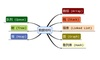数据结构 订阅